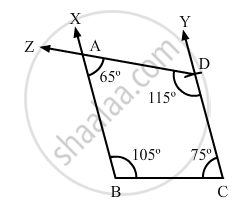Share

# Construct a Quadrilateral Abcd, Where ∠A = 65°, ∠B = 105°, ∠C = 75° Bc = 5.7 Cm and Cd = 6.8 Cm. - Mathematics

Course
ConceptConstructing a Quadrilateral When Two Adjacent Sides and Three Angles Are Known

#### Question

Construct a quadrilateral ABCD, where ∠A = 65°, ∠B = 105°, ∠C = 75° BC = 5.7 cm and CD = 6.8 cm.

#### Solution

$\text { We know that the sum of all the angles in a quadrilateral is 360 } .$

$\text { i . e} . , \angle A + \angle B + \angle C + \angle D = 360^\circ$

$\Rightarrow \angle D = 115^\circ$

Steps of Construction:

Step I: Draw BC = 5 . 7 cm .

$\text { Step II: Construct } \angle XBC = 105^\circ\text { at B and } \angle BCY = 105^\circ at C .$

Step III : With C as the centre and radius 6 . 8 cm, cut off CD = 6 . 8 cm .

$\text { Step IV: At D, draw } \angle CDZ = 115^\circ \text { such that it meets BY at A } .$